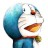# 杰拉斯的博客

## JavaScript编程风格杰拉斯 | 时间：2012-05-05, Sat | 15,878 views

Douglas CrockfordJavascript权威，Json格式就是他的发明。block

{

...

}

block {

...

}

return

{

key:value;

};

return {

key : value;

};

foo (bar)

return(a+b);

if(a === 0) {...}

function foo (b) {...}

function(x) {...}var a = 1

var a = 1;

x = y

(function (){

...

})();

x = y(function (){...})();

with可以减少代码的书写，但是会造成混淆。

with (o) {

foo = bar;

}

o.foo = bar;

o.foo = o.bar;

foo = bar;

foo = o.bar;Javascript有两个表示"相等"的运算符："相等"（==）和"严格相等"（===）。

0 == ''// true

1 == true // true

2 == true // false

0 == '0' // true

false == 'false' // false

false == '0' // true

" \t\r\n " == 0 // true

a = b;

if (a) {...}

if (a = b) {...}

if （a === b）{...}

var a = b = 0;

var a = 0, b = 0;

b = 0;

var a = b;Javascript会自动将变量声明"提升"（hoist）到代码块（block）的头部。

if (!o) {

var o = {};

}

var o;

if (!o) {

o = {};

}

for (var i ...) {...}

var i;

for (i ...) {...,}

Javascript最大的语法缺点，可能就是全局变量对于任何一个代码块，都是可读可写。这对代码的模块化和重复使用，非常不利。Javascript使用new命令，从建构函数生成一个新对象。

var o = new myObject();

++x

x += 1;

++x;

++x;

x += 2;

if (a) b(); c();

if (a) { b(); c();}

if (a) { b();} c();

（完）